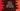# Python modulo explanation with example## Python Modulo operation :

Python modulo operation is used to find out the remainder of a division. Percent symbol % is used for modulo. It is also called modulo operator. In this post, I will quickly explain you how to use python modulo operator with different examples.

### Syntax of modulo :

The syntax of modulo is defined as below :

``x % y``

It will return the remainder of the division x/y. The operands can be an integer or floating point value.

### Example of modulo :

In this example, we are taking two numbers as inputs and calculating the modulo :

``````first_number = int(input("Enter the first number : "))
second_number = int(input("Enter the second number : "))

print("first_number % second_number : {}".format(first_number % second_number))``````

### Sample Outputs :

``````Enter the first number : 123
Enter the second number : 10
first_number % second_number : 3

Enter the first number : 100
Enter the second number : 10
first_number % second_number : 0

Enter the first number : 143
Enter the second number : 8
first_number % second_number : 7``````

## Find out all numbers between 1 to 100 divisible by 6 :

We can use modulo to find out if a number is divisible by any other number or not. If a % b is 0, it means that a is divisible by b. Using this concept, we can print all numbers divisible by a specific number in a range. For example, the below example prints all numbers divisible by 6 between 1 to 100 :

``````for i in range(1, 100):
if (i % 6 == 0):
print(i)``````

### Output :

``````6
12
18
24
30
36
42
48
54
60
66
72
78
84
90
96``````